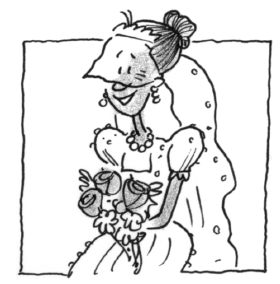### Home > CALC3RD > Chapter Ch7 > Lesson 7.1.2 > Problem7-22

7-22.

THE WEDDING CAKE, Part One

Kiki is ordering a wedding cake for her upcoming reception. She is considering a four-layer circular cake, where the diameters are $16$, $12$, $8$, and $4$ inches. Each layer will be $4$ inches tall.

1. Write a Riemann sum to approximate the volume of Kiki’s cake.

Recall that a Riemann sum is a sum of the area of many rectangles.
Sigma notation is a tool to concisely write that sum, but it is not essential that you use sigma notation.

2. The bakery has informed Kiki that she should have approximately $7$ in3 of cake per guest at the reception. She expects $200$ guests at her wedding. Will she have enough cake?

In order for Kiki to have enough cake to feed her guests, the total volume of all $4$ layers of cake should exceed $7\left(200\right) = 1400$ cubic inches.